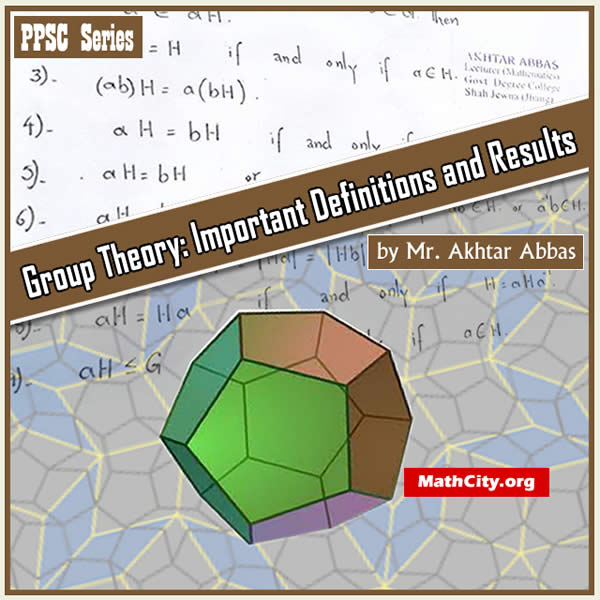# Group Theory: Important Definitions and ResultsThese notes are made and shared by Mr. Akhtar Abbas. We are really very thankful to him for providing these notes and appreciates his effort to publish these notes on MathCity.org. These notes contains important definitions with examples and related theorem, which might be helpful to prepare interviews or any other written test after graduation like PPSC, FPSC or etc.

 Name Group Theory: Important Definitions and Results Mr. Akhtar Abbas 27 pages PDF (see Software section for PDF Reader) 2.21 MB
• A non-empty set $G$ with binary operation * is called group if the binary operation * is associative and
• (1) for all $a\in G$, $\exists$ $e\in G$ s.t $a\text{*} e= e\text{*} a =$
• (2) for each $a\in G$, $\exists$ $a^{-1}\in G$ s.t $a\text{*} a^{-1}=a^{-1}\text{*} a =e$.
• In a group $G$, there is only one identity element.
• In a group $G$, the inverse of the element is unique.
• Every element of $A_n$ is a product of 3-cycles, $n\geq 3$.

Please click on View Online to see inside the PDF.